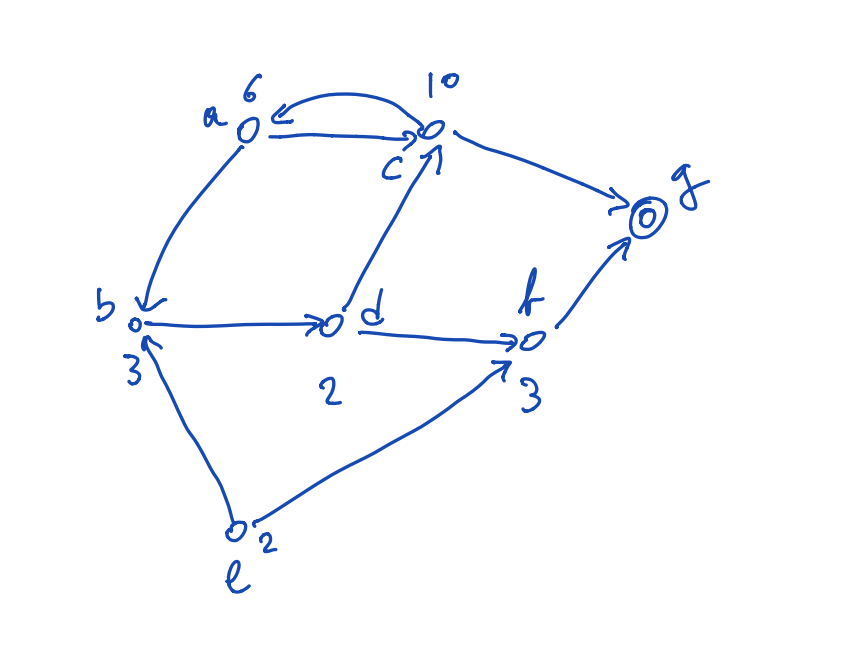## Homework13 Finding the Cheapest Path查找最便宜路径_美国留学生作业assignment## Problem Setting

You are given a graph (?,?)(V,E), where each vertex ??x∈V has a set of successors ?(?)s(x). There is one goal vertex ??g∈V, that one must try to reach. The cost of visiting a vertex ??x∈V is ?(?)>0c(x)>0.

The goal of the homework is to find, from every graph vertex ??x∈V, the path to reach the goal ??g∈V with minumum cost, and to compute for each vertex ??x∈V its value ?(?)v∗(x), which corresponds to the minimum cost of reaching the goal.

You can compute ?(?)v∗(x) for every ??x∈V via dynamic programming. First, you set:

?(?)={0+if ?=?;if ??.(1)v(x)={0if x=g;+∞if x≠g.(1)

Basically, this encodes the fact that if you are at ?g, you are at the goal, and you pay nothing. If you are not at ?g, as we have not yet explored any path, the cost is infinite, as you don’t know how to go to ?g (yet) .

Then, for every ??x≠g, you update the cost ?(?)v(x) of ?x via:

?(?):=?(?)+min??(?)?(?).(2)v(x):=c(x)+miny∈s(x)v(y).(2)

This because, to reach ?g from ??x≠g, one must first pay the price ?(?)c(x) of being at ?x, and then one must go to a successor ?y of ?g. If the cost of then reaching ?g from ?y is ?(?)v(y), the total cost ?(?)v(x) from ?x is ?(?)+?(?)c(x)+v(y). If ?x has many successors, it is convenient to choose the successor with minimum cost: hence the minmin in the above equation.

So one way of solving the problem is this.

Initially, set ?(?)v(x) via (1). Then, repeat:

• Update the value ?(?)v(x) at each ??x≠g via (2)

Until nothing changes when the update is done.

The last part of the problem consists in finding a minimum-cost path to the goal. But this is easy to do: all you need to do is, when you update the costs ?(?)v(x) of a vertex via (2), you remember which successor ??(?)y∈s(x) gave you the minimum value (if there is more than one ??(?)y∈s(x) that gives you the minimum value, pick one of them at random). Call this the optimal successor ?(?)b(x) (for, “best at ?x“) of ?x:

?(?)=argmin??(?)?(?).(3)b(x)=arg⁡miny∈s(x)v(y).(3)

Then, to reach ?g with minimum cost, you simply take the edge from ?x to ?1=?(?)y1=b(x), then to ?2=?(?1)y2=b(y1), and so forth, until you reach ?g. That is, you do not need to remember from each state its path to ?g. You just need to remember the best successor of every state: following this chain of best successors will lead you to ?g with minimum cost.

Here is the representation of a vertex, including its cost ?()c(⋅), value ?()v(⋅), and best successor ?()b(⋅).

class Vertex(object):
"""This represents a vertex of the graph."""

def __init__(self, cost, name=""):
assert cost > 0
assert len(name) > 0
self.cost = cost
self.name = name
# This will be computed later
self.value = None
self.best_successor = None

def __hash__(self):
return hash(self.name)

def __repr__(self):
return "{}(c={},v={})".format(self.name, self.cost, self.value)


We define infinity, to implement (1). Yes, you can represent infinity in Python.

INFINITE = float("inf")

## Question 1.

Here is our definition of the graph. In it, you need to complete the compute_values and best_path methods.

• The compute_values method should perform the computation of the minimum cost for reaching the goal from each vertex, and should update x.value and x.best_successor for every vertex.
• The best_path method should return the best path from a vertex to the goal, including both the initial vertex, and the goal vertex.
from collections import defaultdict

class Graph(object):

def __init__(self):
self.vertices = set()
self.successors = defaultdict(set)
self.goal = None # We will set this later.

"""Adds a vertex x, with a specified set of successors."""
# First, we want to make sure that both x and its successors are in the set
# of graph vertices.
self.vertices.update(successors)
# Then, we want to keep track of the successors of x.
self.successors[x] = successors

"""Adding a goal is similar to adding a vertex, except that the goal has
no successors (you have already arrived!). """
self.goal = x

def compute_values(self):
"""This function should compute the values x.value for each vertex
x of the graph, along with the best successor x.best_successor of each
vertex."""
pass
# This can be done in about a dozen lines of code.

def best_path(self, x):
"""This function should output a list of vertices, starting at x,
and ending at the goal vertex, that consists of a minimum-cost path
to the goal."""
pass
# This can be done in 5-6 lines of code.

### 公众号：李兴球Python